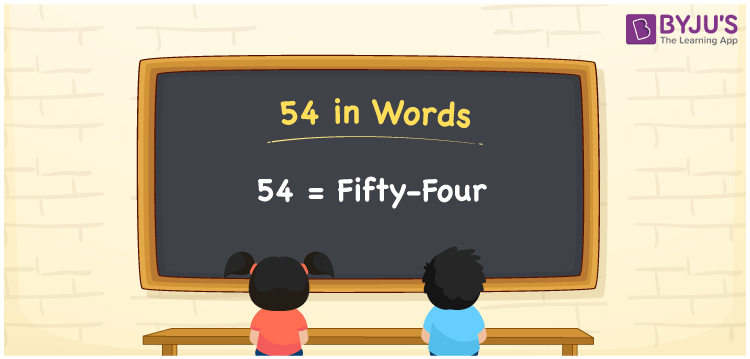# 54 in Words

In words, 54 equals “Fifty-four“. 54 is four more than fifty. 54 is a number that signifies a value or a count equivalent to the objects specified. For example, Ramya purchased a pen for Rs. 54. We can write the same sentence in words as Ramya purchased a pen for Rs. Fifty-four. As a result, students must learn numbers in words to express them. In this article, we will learn how to write 54 in words.

 54 in Words: Fifty-four. Fifty-four in Numerical Form: 54.

## 54 in English Words## How to Write 54 in Words?

To write the number 54 in words, first, determine the place value of each digit of the given number. Following is the place value table for the number 54.

 Tens Ones 5 4

The expanded form for 54 is as follows.

= 5 × Ten + 4 × One

= 5 × 10 + 4 × 1

= 50 + 4

= 54

= Fifty-four

Hence, 54 in words is Fifty-four.

54 in words – Fifty-four

Is 54 an odd number? – No

Is 54 an even number? – Yes

Is 54 a perfect square number? – No

Is 54 a perfect cube number? – No

Is 54 a prime number? – No

Is 54 a composite number? – Yes

## Frequently Asked Questions on 54 in Words

Q1

### Write 54 in words.

54 in words is fifty-four.

Q2

### Simplify 60 – 6, and express in words.

Simplifying 60 – 6, we get 54. Hence, 54 in words is fifty-four.

Q3

### How to write fifty-four in numbers?

Fifty-four in numbers is 54.